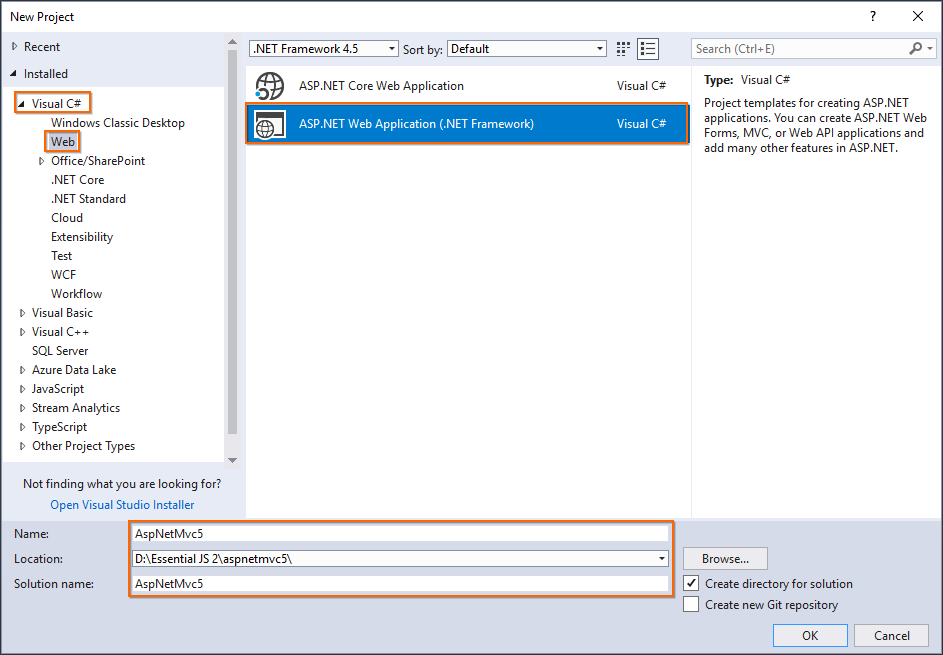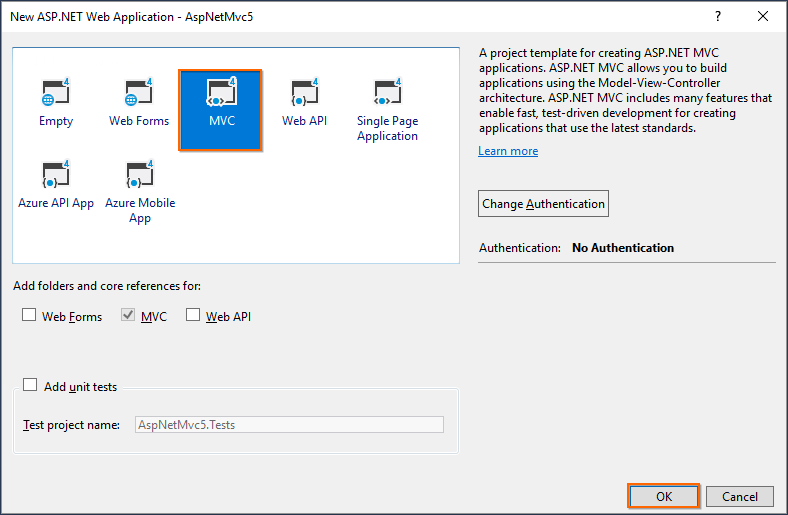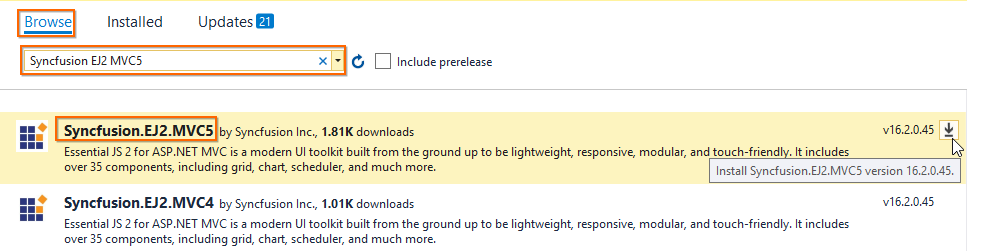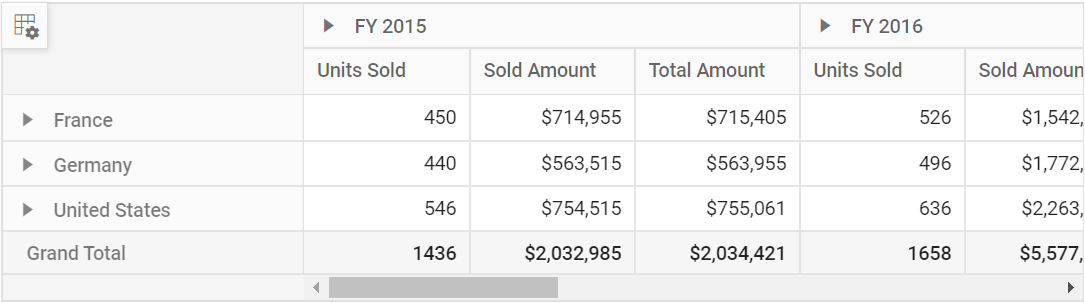Search results

# Getting started with ASP.NET MVC Pivot Table control

Starting with v16.2.0.x, if you reference Syncfusion assemblies from trial setup or from the NuGet feed, you also have to include a license key in your projects. Please refer to this link to know about registering Syncfusion license key in your ASP.NET MVC application to use our components.

## Prerequisites

To get start with ASP.NET MVC application, need to ensure the following software to be installed on the machine.

1. .NET Framework 4.5 and above.
2. ASP.NET MVC 4 or ASP.NET MVC 5
3. Visual Studio

## Preparing ASP.NET MVC application

Follow below steps to create ASP.NET MVC Application.

Step 1: Choose File > New > Project… in the Visual Studio menu bar.Step 2: Select Installed > Visual C# > Web and choose the required .NET Framework in the drop-down.

Step 3: Select ASP.NET Web Application (.NET Framework) and change the application name, and then click OK.

The Essential JS 2 supports 4.5+ .NET Framework in the ASP.NET MVC application. i.e. The minimum target framework is 4.5 for Syncfusion ASP.NET MVC (Essential JS 2).Step 4: Choose MVC and then click OK. Now, the MVC web application project is created with default ASP.NET MVC template.## Configure Essential JS 2 in the application

Step 1: Add the `Syncfusion.EJ2.MVC5` NuGet package to the new application by using the Nuget Package Manager. Right-click the project and select Manage NuGet Packages….Step 2: Search the `Syncfusion EJ2 MVC5` keyword in the Browse tab and install Syncfusion.EJ2.MVC5 NuGet package in the application.The Essential JS 2 MVC5 NuGet package will be included in the project, after the installation process is completed.

Note: The Syncfusion.EJ2.MVC5 NuGet package has dependencies, `Newtonsoft.Json` for JSON serialization and `Syncfusion.Licensing` for validating Syncfusion license key.

Step 3: Open `~/Views/Web.config` file and add the `Syncfusion.EJ2` namesapce reference to the `<system.web.webPages.razor>` element and `Syncfusion.EJ2` assembly reference to `<system.web>` element.

``````<configuration>
....
....
<system.web.webPages.razor>
....
....
<pages pageBaseType="System.Web.Mvc.WebViewPage">
<namespaces>
....
....
</namespaces>
</pages>
</system.web.webPages.razor>
....
....
<system.web>
<compilation>
<assemblies>
....
....
</assemblies>
</compilation>
</system.web>
</configuration>``````

Step 4: Add the client-side resources through CDN in the `<head>` element of `~/Views/Shared/_Layout.cshtml` layout page.

``````    <head>
....
....

<!-- Syncfusion Essential JS 2 Styles -->

<!-- Syncfusion Essential JS 2 Scripts -->
<script src="https://cdn.syncfusion.com/ej2/dist/ej2.min.js"></script>

Step 5: Add the Essential JS 2 Script Manager at the end of `<body>` element in the `~/Views/Shared/_Layout.cshtml` layout page.

``````    <body>
....
....
<!-- Syncfusion Essential JS 2 ScriptManager -->
@Html.EJS().ScriptManager()
</body>``````

## Browser compatibility

Polyfills are required to use the Pivot Table in Internet Explorer 11 browser. Refer the documentation for more details.

## Adding component to the application

• Add the below code to your `Index.cshtml` view page which is present under `Views/Home` folder, to initialize the pivot table component.
• The Pivot Table component further needs to be populated with an appropriate data source. For illustration purpose, a collection of objects mentioning the sales details of certain products over a period and region has been prepared. This sample data is assigned to the pivot table component through `DataSource` property under `PivotViewDataSourceSettings` class.
razor
initial.cs
``@Html.EJS().PivotView("PivotView").Height("300").DataSourceSettings(dataSource => dataSource.DataSource((IEnumerable<object>)ViewBag.DataSource)).Render()``
``````public ActionResult Index()
{
var data = GetPivotData();
ViewBag.DataSource = data;
return View();
}

public List<PivotData> GetPivotData()
{
List<PivotData> pivotData = new List<PivotData>();
pivotData.Add(new PivotData { Sold = 31, Amount = 52824, Country = "France", Products = "Mountain Bikes", Year = "FY 2015", Quarter = "Q1" });
pivotData.Add(new PivotData { Sold = 51, Amount = 86904, Country = "France", Products = "Mountain Bikes", Year = "FY 2015", Quarter = "Q2" });
pivotData.Add(new PivotData { Sold = 90, Amount = 153360, Country = "France", Products = "Mountain Bikes", Year = "FY 2015", Quarter = "Q3" });
pivotData.Add(new PivotData { Sold = 25, Amount = 42600, Country = "France", Products = "Mountain Bikes", Year = "FY 2015", Quarter = "Q4" });
pivotData.Add(new PivotData { Sold = 27, Amount = 46008, Country = "France", Products = "Mountain Bikes", Year = "FY 2016", Quarter = "Q1" });
pivotData.Add(new PivotData { Sold = 49, Amount = 83496, Country = "France", Products = "Mountain Bikes", Year = "FY 2016", Quarter = "Q2" });
pivotData.Add(new PivotData { Sold = 95, Amount = 161880, Country = "France", Products = "Mountain Bikes", Year = "FY 2016", Quarter = "Q3" });
pivotData.Add(new PivotData { Sold = 67, Amount = 114168, Country = "France", Products = "Mountain Bikes", Year = "FY 2016", Quarter = "Q4" });
pivotData.Add(new PivotData { Sold = 75, Amount = 127800, Country = "France", Products = "Mountain Bikes", Year = "FY 2017", Quarter = "Q1" });
pivotData.Add(new PivotData { Sold = 67, Amount = 114168, Country = "France", Products = "Mountain Bikes", Year = "FY 2017", Quarter = "Q2" });
pivotData.Add(new PivotData { Sold = 69, Amount = 117576, Country = "France", Products = "Mountain Bikes", Year = "FY 2017", Quarter = "Q3" });
pivotData.Add(new PivotData { Sold = 90, Amount = 153360, Country = "France", Products = "Mountain Bikes", Year = "FY 2017", Quarter = "Q4" });
pivotData.Add(new PivotData { Sold = 16, Amount = 27264, Country = "France", Products = "Mountain Bikes", Year = "FY 2018", Quarter = "Q1" });
pivotData.Add(new PivotData { Sold = 83, Amount = 124422, Country = "France", Products = "Road Bikes", Year = "FY 2015", Quarter = "Q1" });
pivotData.Add(new PivotData { Sold = 57, Amount = 85448, Country = "France", Products = "Road Bikes", Year = "FY 2015", Quarter = "Q2" });
pivotData.Add(new PivotData { Sold = 20, Amount = 29985, Country = "France", Products = "Road Bikes", Year = "FY 2015", Quarter = "Q3" });
pivotData.Add(new PivotData { Sold = 67, Amount = 70008, Country = "France", Products = "Road Bikes", Year = "FY 2016", Quarter = "Q1" });
pivotData.Add(new PivotData { Sold = 89, Amount = 60496, Country = "France", Products = "Road Bikes", Year = "FY 2016", Quarter = "Q2" });
pivotData.Add(new PivotData { Sold = 75, Amount = 801880, Country = "France", Products = "Road Bikes", Year = "FY 2016", Quarter = "Q3" });
pivotData.Add(new PivotData { Sold = 57, Amount = 204168, Country = "France", Products = "Road Bikes", Year = "FY 2016", Quarter = "Q4" });
pivotData.Add(new PivotData { Sold = 75, Amount = 737800, Country = "France", Products = "Road Bikes", Year = "FY 2017", Quarter = "Q1" });
pivotData.Add(new PivotData { Sold = 87, Amount = 884168, Country = "France", Products = "Road Bikes", Year = "FY 2017", Quarter = "Q2" });
pivotData.Add(new PivotData { Sold = 39, Amount = 729576, Country = "France", Products = "Road Bikes", Year = "FY 2017", Quarter = "Q3" });
pivotData.Add(new PivotData { Sold = 90, Amount = 38860, Country = "France", Products = "Road Bikes", Year = "FY 2017", Quarter = "Q4" });
pivotData.Add(new PivotData { Sold = 93, Amount = 139412, Country = "France", Products = "Road Bikes", Year = "FY 2015", Quarter = "Q4" });
pivotData.Add(new PivotData { Sold = 51, Amount = 92824, Country = "Germany", Products = "Mountain Bikes", Year = "FY 2015", Quarter = "Q1" });
pivotData.Add(new PivotData { Sold = 61, Amount = 76904, Country = "Germany", Products = "Mountain Bikes", Year = "FY 2015", Quarter = "Q2" });
pivotData.Add(new PivotData { Sold = 70, Amount = 43360, Country = "Germany", Products = "Mountain Bikes", Year = "FY 2015", Quarter = "Q3" });
pivotData.Add(new PivotData { Sold = 85, Amount = 62600, Country = "Germany", Products = "Mountain Bikes", Year = "FY 2015", Quarter = "Q4" });
pivotData.Add(new PivotData { Sold = 97, Amount = 86008, Country = "Germany", Products = "Mountain Bikes", Year = "FY 2016", Quarter = "Q1" });
pivotData.Add(new PivotData { Sold = 69, Amount = 93496, Country = "Germany", Products = "Mountain Bikes", Year = "FY 2016", Quarter = "Q2" });
pivotData.Add(new PivotData { Sold = 45, Amount = 301880, Country = "Germany", Products = "Mountain Bikes", Year = "FY 2016", Quarter = "Q3" });
pivotData.Add(new PivotData { Sold = 77, Amount = 404168, Country = "Germany", Products = "Mountain Bikes", Year = "FY 2016", Quarter = "Q4" });
pivotData.Add(new PivotData { Sold = 15, Amount = 137800, Country = "Germany", Products = "Mountain Bikes", Year = "FY 2017", Quarter = "Q1" });
pivotData.Add(new PivotData { Sold = 37, Amount = 184168, Country = "Germany", Products = "Mountain Bikes", Year = "FY 2017", Quarter = "Q2" });
pivotData.Add(new PivotData { Sold = 49, Amount = 89576, Country = "Germany", Products = "Mountain Bikes", Year = "FY 2017", Quarter = "Q3" });
pivotData.Add(new PivotData { Sold = 40, Amount = 33360, Country = "Germany", Products = "Mountain Bikes", Year = "FY 2017", Quarter = "Q4" });
pivotData.Add(new PivotData { Sold = 96, Amount = 77264, Country = "Germany", Products = "Mountain Bikes", Year = "FY 2018", Quarter = "Q1" });
pivotData.Add(new PivotData { Sold = 23, Amount = 24422, Country = "Germany", Products = "Road Bikes", Year = "FY 2015", Quarter = "Q1" });
pivotData.Add(new PivotData { Sold = 67, Amount = 75448, Country = "Germany", Products = "Road Bikes", Year = "FY 2015", Quarter = "Q2" });
pivotData.Add(new PivotData { Sold = 70, Amount = 52345, Country = "Germany", Products = "Road Bikes", Year = "FY 2015", Quarter = "Q3" });
pivotData.Add(new PivotData { Sold = 13, Amount = 135612, Country = "Germany", Products = "Road Bikes", Year = "FY 2015", Quarter = "Q4" });
pivotData.Add(new PivotData { Sold = 57, Amount = 90008, Country = "Germany", Products = "Road Bikes", Year = "FY 2016", Quarter = "Q1" });
pivotData.Add(new PivotData { Sold = 29, Amount = 90496, Country = "Germany", Products = "Road Bikes", Year = "FY 2016", Quarter = "Q2" });
pivotData.Add(new PivotData { Sold = 45, Amount = 301880, Country = "Germany", Products = "Road Bikes", Year = "FY 2016", Quarter = "Q3" });
pivotData.Add(new PivotData { Sold = 77, Amount = 404168, Country = "Germany", Products = "Road Bikes", Year = "FY 2016", Quarter = "Q4" });
pivotData.Add(new PivotData { Sold = 15, Amount = 137800, Country = "Germany", Products = "Road Bikes", Year = "FY 2017", Quarter = "Q1" });
pivotData.Add(new PivotData { Sold = 37, Amount = 184168, Country = "Germany", Products = "Road Bikes", Year = "FY 2017", Quarter = "Q2" });
pivotData.Add(new PivotData { Sold = 99, Amount = 829576, Country = "Germany", Products = "Road Bikes", Year = "FY 2017", Quarter = "Q3" });
pivotData.Add(new PivotData { Sold = 80, Amount = 38360, Country = "Germany", Products = "Road Bikes", Year = "FY 2017", Quarter = "Q4" });
pivotData.Add(new PivotData { Sold = 91, Amount = 67824, Country = "United States", Products = "Mountain Bikes", Year = "FY 2015", Quarter = "Q1" });
pivotData.Add(new PivotData { Sold = 81, Amount = 99904, Country = "United States", Products = "Mountain Bikes", Year = "FY 2015", Quarter = "Q2" });
pivotData.Add(new PivotData { Sold = 70, Amount = 49360, Country = "United States", Products = "Mountain Bikes", Year = "FY 2015", Quarter = "Q3" });
pivotData.Add(new PivotData { Sold = 65, Amount = 69600, Country = "United States", Products = "Mountain Bikes", Year = "FY 2015", Quarter = "Q4" });
pivotData.Add(new PivotData { Sold = 57, Amount = 90008, Country = "United States", Products = "Mountain Bikes", Year = "FY 2016", Quarter = "Q1" });
pivotData.Add(new PivotData { Sold = 29, Amount = 90496, Country = "United States", Products = "Mountain Bikes", Year = "FY 2016", Quarter = "Q2" });
pivotData.Add(new PivotData { Sold = 85, Amount = 391880, Country = "United States", Products = "Mountain Bikes", Year = "FY 2016", Quarter = "Q3" });
pivotData.Add(new PivotData { Sold = 97, Amount = 904168, Country = "United States", Products = "Mountain Bikes", Year = "FY 2016", Quarter = "Q4" });
pivotData.Add(new PivotData { Sold = 85, Amount = 237800, Country = "United States", Products = "Mountain Bikes", Year = "FY 2017", Quarter = "Q1" });
pivotData.Add(new PivotData { Sold = 77, Amount = 384168, Country = "United States", Products = "Mountain Bikes", Year = "FY 2017", Quarter = "Q2" });
pivotData.Add(new PivotData { Sold = 99, Amount = 829576, Country = "United States", Products = "Mountain Bikes", Year = "FY 2017", Quarter = "Q3" });
pivotData.Add(new PivotData { Sold = 80, Amount = 38360, Country = "United States", Products = "Mountain Bikes", Year = "FY 2017", Quarter = "Q4" });
pivotData.Add(new PivotData { Sold = 76, Amount = 97264, Country = "United States", Products = "Mountain Bikes", Year = "FY 2018", Quarter = "Q1" });
pivotData.Add(new PivotData { Sold = 53, Amount = 94422, Country = "United States", Products = "Road Bikes", Year = "FY 2015", Quarter = "Q1" });
pivotData.Add(new PivotData { Sold = 90, Amount = 45448, Country = "United States", Products = "Road Bikes", Year = "FY 2015", Quarter = "Q2" });
pivotData.Add(new PivotData { Sold = 29, Amount = 92345, Country = "United States", Products = "Road Bikes", Year = "FY 2015", Quarter = "Q3" });
pivotData.Add(new PivotData { Sold = 67, Amount = 235612, Country = "United States", Products = "Road Bikes", Year = "FY 2015", Quarter = "Q4" });
pivotData.Add(new PivotData { Sold = 97, Amount = 90008, Country = "United States", Products = "Road Bikes", Year = "FY 2016", Quarter = "Q1" });
pivotData.Add(new PivotData { Sold = 79, Amount = 90496, Country = "United States", Products = "Road Bikes", Year = "FY 2016", Quarter = "Q2" });
pivotData.Add(new PivotData { Sold = 95, Amount = 501880, Country = "United States", Products = "Road Bikes", Year = "FY 2016", Quarter = "Q3" });
pivotData.Add(new PivotData { Sold = 97, Amount = 104168, Country = "United States", Products = "Road Bikes", Year = "FY 2016", Quarter = "Q4" });
pivotData.Add(new PivotData { Sold = 95, Amount = 837800, Country = "United States", Products = "Road Bikes", Year = "FY 2017", Quarter = "Q1" });
pivotData.Add(new PivotData { Sold = 87, Amount = 684168, Country = "United States", Products = "Road Bikes", Year = "FY 2017", Quarter = "Q2" });
pivotData.Add(new PivotData { Sold = 109, Amount = 29576, Country = "United States", Products = "Road Bikes", Year = "FY 2017", Quarter = "Q3" });
return pivotData;
}

public class PivotData
{
public int Sold { get; set; }
public double Amount { get; set; }
public string Country { get; set; }
public string Products { get; set; }
public string Year { get; set; }
public string Quarter { get; set; }
}``````

## Adding fields to row, column, value and filter axes

Now that pivot table is initialized and assigned with sample data, will further move to showcase the component by organizing appropriate fields in row, column, value and filter axes.

In `PivotViewDataSourceSettings` class, four major axes - `Rows`, `Columns`, `Values` and `Filters` plays a vital role in defining and organizing fields from the bound data source, to render the entire pivot table component in a desired format.

`Rows` – Collection of fields that needs to be displayed in row axis of the pivot table.

`Columns` – Collection of fields that needs to be displayed in column axis of the pivot table.

`Values` – Collection of fields that needs to be displayed as aggregated numeric values in the pivot table.

`Filters` - Collection of fields that would act as master filter over the data bound in row, column and value axes of the pivot table.

In-order to define each field in the respective axis, the following basic properties should be set.

• `Name`: It allows to set the field name from the bound data source. It’s casing should match exactly like in the data source and if not set properly, the pivot table will not be rendered.
• `Caption`: It allows to set the field caption, which is the alias name of the field that needs to be displayed in the pivot table.
• `Type`: It allows to set the summary type of the field. By default, SummaryTypes.Sum is applied.

In this illustration, “Year” and “Quarter” are added in column, “Country” and “Products” in row, and “Sold” and “Amount” in value section respectively.

razor
fields.cs
``````@Html.EJS().PivotView("PivotView").Height("300").DataSourceSettings(dataSource => dataSource.DataSource((IEnumerable<object>)ViewBag.DataSource).ExpandAll(false).EnableSorting(true)
.Rows(rows =>
{
}).Columns(columns =>
{
}).Values(values =>
{
})).Render()``````
``````public ActionResult Index()
{
var data = GetPivotData();
ViewBag.DataSource = data;
return View();
}``````

## Applying formatting to a value field

Formatting defines a way in which values should be displayed. For example, format “C” denotes the values should be displayed in currency pattern. To do so, define the `FormatSetting` class with its `Name` and `Format` properties and add it to `PivotViewFormatSettings`. In this illustration, the `Name` property is set as Amount, a field from value section and its format is set as currency. Likewise, we can set format for other value fields as well and add it to `PivotViewFormatSettings`.

Only fields from value section, which is in the form of numeric data values are applicable for formatting.

razor
format.cs
``````@Html.EJS().PivotView("PivotView").Height("300").DataSourceSettings(dataSource => dataSource.DataSource((IEnumerable<object>)ViewBag.DataSource).ExpandAll(false).EnableSorting(true)
.FormatSettings(formatsettings =>
{
}).Rows(rows =>
{
}).Columns(columns =>
{
}).Values(values =>
{
})).Render()``````
``````public ActionResult Index()
{
var data = GetPivotData();
ViewBag.DataSource = data;
return View();
}``````

## Enable Grouping Bar

The grouping bar feature automatically populates fields from the bound data source and allows end users to drag fields between different axes such as columns, rows, values, and filters, and alter pivot table at runtime. It also provides option to sort, filter and remove fields. It can be enabled by setting the `ShowGroupingBar` property to true.

razor
groupingbar.cs
``````@Html.EJS().PivotView("PivotView").Width("100%").Height("300").DataSourceSettings(dataSource => dataSource.DataSource((IEnumerable<object>)ViewBag.DataSource).ExpandAll(false).EnableSorting(true).AllowLabelFilter(true).AllowValueFilter(true)
.FormatSettings(formatsettings =>
{
}).Rows(rows =>
{
}).Columns(columns =>
{
}).Values(values =>
{
})).ShowGroupingBar(true).Render()``````
``````public ActionResult Index()
{
var data = GetPivotData();
ViewBag.DataSource = data;
return View();
}``````

## Enable Pivot Field List

The field list allows to add or remove fields and also rearrange the fields between different axes, including column, row, value, and filter along with filter and sort options dynamically at runtime. It can be enabled by setting the `ShowFieldList` property to true.

razor
fieldlist.cs
``````@Html.EJS().PivotView("PivotView").Height("300").DataSourceSettings(dataSource => dataSource.DataSource((IEnumerable<object>)ViewBag.DataSource).ExpandAll(false).EnableSorting(true).AllowLabelFilter(true).AllowValueFilter(true)
.FormatSettings(formatsettings =>
{
}).Rows(rows =>
{
}).Columns(columns =>
{
}).Values(values =>
{
})).ShowFieldList(true).Render()``````
``````public ActionResult Index()
{
var data = GetPivotData();
ViewBag.DataSource = data;
return View();
}``````

## Calculated field

The calculated field feature allows user to insert or add a new calculated field based on the available fields from the bound data source using basic arithmetic operators. The calculated field can be included in pivot table using the `CalculatedFieldSetting` class from code behind. Or else, calculated fields can be added at run time through the built-in dialog by just setting the `AllowCalculatedField` property to true in pivot table. You will see a button enabled in the Field List UI automatically to invoke the calculated field dialog and perform necessary operation.

Calculated field is applicable only for value fields.

razor
calculatedfield.cs
``````@{var amount = "\"" + "Sum(Amount)" + "\"";}
@{var sold = "\"" + "Sum(Sold)" + "\"";}
@{ var totalPrice = amount + "+" + sold;}

@Html.EJS().Button("calculated-field-btn").Content("Calculated Field").IsPrimary(true).Render()
@Html.EJS().PivotView("PivotView").Height("300").DataSourceSettings(dataSource => dataSource.DataSource((IEnumerable<object>)ViewBag.DataSource).ExpandAll(false).EnableSorting(true)
.FormatSettings(formatsettings =>
{
}).Rows(rows =>
{
}).Columns(columns =>
{
}).Values(values =>
{
}).CalculatedFieldSettings(calculatedfieldsettings =>
{
})).AllowCalculatedField(true).Render()

<script>
var pivotObj = document.getElementById("PivotView").ej2_instances;
pivotObj.createCalculatedFieldDialog();
});
</script>``````
``````public ActionResult Index()
{
var data = GetPivotData();
ViewBag.DataSource = data;
return View();
}``````

Output be like the below.